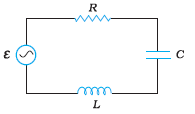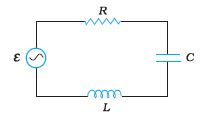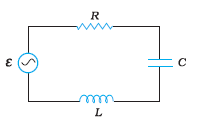## Filters

Sort by :
Clear All
Q

Q7.18 (a) A circuit containing a  inductor and a   capacitor in series is connected to a   supply. The resistance of the circuit is negligible. Obtain the current amplitude and rms values.

The inductance of the inductor  The capacitance of the capacitor  Voltage supply  Frequency of voltage supply . Here, we have   Impedance   Now, Current in the circuit will be  where,  The negative sign is just a matter of the direction of current.so, here   But, since the value of R is zero(since our circuit have only L and C) Hence   Now,  RMS value of this current:  .

Q7.17  Keeping the source frequency equal to the resonating frequency of the series  circuit, if the three elements, and  are arranged in parallel, show that the total current in the parallel  circuit is minimum at this frequency. Obtain the current rms value in each branch of the circuit for the elements and source specified in Exercise 7.11 for this frequency.

As we know, in the case of a parallel RLC circuit:   The current will be minimum when Which is also the condition of natural frequency. Hence the total current is minimum when source frequency is equal to the natural frequency. RMS value of current in R  RMS value in Inductor RMS value in capacitor Capacitor current and inductor current will cancel out each other so the current flowing in...

Q7.16  Obtain the answers to (a) and (b) in Exercise 7.15 if the circuit is connected to a   supply? Hence, explain the statement that a capacitor is a conductor at very high frequencies. Compare this behaviour with that of a capacitor in a dc circuit after the steady state.

Given, The capacitance of the capacitor  The resistance of the circuit  Voltage supply  Frequency of voltage supply  The maximum current in the circuit Hence maximum current in the circuit is 3.9A. b) In the case of capacitor, we have  So, So the time lag between max voltage and max current is : At high frequencies,  tends to zero. which indicates capacitor acts as a conductor at high...

Q 7.15 (b)   capacitor in series with a resistance is connected to a ,    supply. What is the time lag between the current maximum and the voltage maximum?

In the case of a capacitor, we have  So, So the time lag between max voltage and the max current is :

Q7.15 (a)    capacitor in series with a  resistance is connected to a , supply.
What is the maximum current in the circuit?

Given, The capacitance of the capacitor  The resistance of the circuit  Voltage supply  Frequency of voltage supply  The maximum current in the circuit Hence maximum current in the circuit is 3.24A.

Q7.14 Obtain the answers (a) to (b) in Exercise 7.13 if the circuit is connected to a high-frequency supply . Hence, explain the statement that at very high frequency, an inductor in a circuit nearly amounts to an open circuit. How does an inductor behave in a dc circuit after the steady state?

Given, The inductance of the coil  the resistance of the coil  Supply voltage  Supply voltage frequency a) Now, as we know peak voltage = (RMS Voltage) Peak voltage  Now,  The impedance of the circuit : Now peak current in the circuit : Hence peak current is  in the circuit. The current in the circuit is very small, which is one of the indications of inductor working as a nearly open circuit...

Q 7.13 (b) A coil of inductance and resistance  is connected to a   ac supply. What is the time lag between the voltage maximum and the current maximum?

Let the voltage  in the circuit be   and  Current in the circuit be  Where  is the phase difference between voltage and current.  V is maximum At t = 0  is maximum At      Hence, the time lag between voltage maximum and the current maximum is  . For phase difference   we have  Hence time lag between the maximum voltage and the maximum current is

Q 7.13 (a)   A coil of inductance and resistance  is connected to a    ac supply. What is the maximum current in the coil?

Given, The inductance of the coil  the resistance of the coil  Supply voltage  Supply voltage frequency Now, as we know peak voltage = (RMS Voltage) Peak voltage    The impedance of the circuit : Now peak current in the circuit : Hence peak current is 1.82A in the circuit.

Q7.12 (e)    An  circuit contains a  inductor and a  capacitor with an initial charge of . The resistance of the circuit is negligible. Let the instant the circuit is closed be .

If a resistor is inserted in the circuit, how much energy is eventually dissipated as heat?

If the resistance is added to the circuit, the whole energy will dissipate as heat eventually. energy will keep moving between the capacitor and inductor with reducing in magnitude in each cycle and eventually all energy will be dissipated.

Q7.12 (d)    An  circuit contains a  inductor and a  capacitor with an initial charge of . The resistance of the circuit is negligible. Let the instant the circuit is closed be .

At what times is the total energy shared equally between the inductor and the capacitor?

The energy will be shared equally when the energy in the capacitor is half of the maximum energy it can store.i.e. From Here, we got So Now, we know the charge on the capacitor, we can calculate time for which From here,   Hence for these times, the total energy will be shared equally between capacitor and inductor.

Q7.12 An  circuit contains a  inductor and a  capacitor with an initial charge of . The resistance of the circuit is negligible.
(C) Let the instant the circuit is closed be .

(ii) completely magnetic (i.e., stored in the inductor)?

The stored energy will we purely magnetic when the pure electrical stored is zero. i.e. when the charge on the capacitor is zero, all energy will be stored in the inductor. So, t for which charge on the capacitor is zero is Hence at these times, the total energy will be purely magnetic.

Q7.12  An  circuit contains a  inductor and a  capacitor withan initial charge of . The resistance of the circuit is negligible. Let the instant the circuit is closed be .

( c)     At what time is the energy stored

(i) completely electrical (i.e., stored in the capacitor)?

at any instant, the charge on the capacitor is: Where time period :   Now, when the total energy is purely electrical, we can say that    this is possible when   Hence Total energy will be purely electrical(stored in a capacitor) at  .

Q7.12 (b)    An  circuit contains a  inductor and a  capacitor with an initial charge of . The resistance of the circuit is negligible. Let the instant the circuit is closed be .

What is the natural frequency of the circuit?

Given, The inductance of the inductor:   The capacitance of the capacitor :   The initial charge on the capacitor: The natural angular  frequency of the circuit: Hence the natural angular frequency of the circuit is . The natural frequency of the circuit: Hence natural frequency of the circuit is 159Hz.

Q7.12  (a) An  circuit contains a  inductor and a  capacitor with an initial charge of . The resistance of the circuit is negligible. Let the instant the circuit is closed be .

What is the total energy stored initially? Is it conserved during oscillations?

Given, The inductance of the inductor:   The capacitance of the capacitor :   The initial charge on the capacitor: Total energy present at the initial moment: Hence initial energy in the circuit is 1J. Since we don't have any power-consuming element like  resistance in the circuit, the energy will be conserved

Q7.11    Figure shows a series LCR circuit connected to a variable frequency  source.

, .

Determine the rms potential drops across the three elements of the circuit. Show that the potential drop across the LC combination is zero at the resonating frequency.Potential difference across any element =  NOW, The potential difference across capacitor: Potential difference across the inductor  The potential difference across Resistor  Potential difference across LC combination Hence at resonating frequency potential difference across LC combination is zero.

Q7.11 (b) Figure  shows a series LCR circuit connected to a variable frequency  source.

, .

(b) Obtain the impedance of the circuit and the amplitude of current at the resonating frequency.Given, Variable frequency supply voltage  = 230V Inductance  Capacitance  Resistance  Now, The impedance of the circuit is    at Resonance Condition : Hence, Impedance at resonance is 40. Now, at resonance condition, impedance is minimum which means current is maximum which will happen when we have a peak voltage, so Current in the Resonance circuit is Given by  Hence amplitude of the current...

Q7.11     Figure shows a series LCR circuit connected to a variable frequency  source.  , .

(a)    Determine the source frequency which drives the circuit in resonance.Given, Variable frequency supply voltage  = 230V Inductance  Capacitance  Resistance  a) Resonance angular frequency in this circuit is given by : Hence this circuit will be in resonance when supply frequency is 50 rad/sec.

Q7.10  A radio can tune over the frequency range of a portion of  broadcast band:    . If its circuit has an effective inductance of , what must be the range of its variable capacitor?

Given,  Range of the frequency in which radio can be tune =  The effective inductance of the Circuit =  Now, As we know,   where  is tuning frequency. For getting the range of the value of a capacitor, let's calculate the two values of the capacitor, one maximum, and one minimum. first, let's calculate the minimum value of capacitance which is the case when tuning frequency = 800KHz. Hence...

Q7.9  A series LCR circuit with   and is connected to a variable-frequency  ac supply. When the frequency of the supply equals the natural frequency of the circuit, what is the average power transferred to the circuit in one complete cycle?

Given, Resistance  Inductance  Capacitance  Voltage supply  At resonance, supply frequency is equal to the natural frequency, and at the natural frequency, the total impedance of the circuit is equal to the resistance of the circuit  as inductive and capacitive reactance cancels each other. in other words, As    Now, Current in the circuit Average Power transferred in the circuit : Hence...

Q7.8  Suppose the initial charge on the capacitor in Exercise 7.7 is  . What is the total energy stored in the circuit initially? What is the total energy at later time?

Given Capacitance   Inductance  Charge on the capacitor   Now, The total energy stored in Capacitor : Total energy later will be same because energy is being shared with capacitor and inductor and none of them loses the energy, they just store it and transfer it.
Exams
Articles
Questions